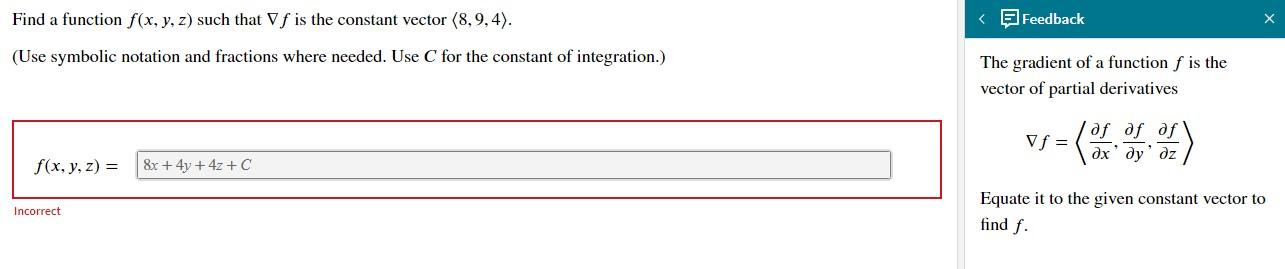# Question Find a function 𝑓(𝑥,𝑦,𝑧) such that ∇𝑓 is the constant vector 〈8,9,4〉. (Use symbolic notation and fractions where needed. Use 𝐶 for the constant of integration.) Find a function f(x, y, z) such that f is the constant vector (8,9,4). < | Feedback (Use symbolic notation and fractions where needed. Use C for the constant of integration.) The gradient of a function f is the vector of partial derivatives af af af Vf= дх dy' дz v=) f(x, y, z) = 8x + 4y + 4z +C Equate it to the given constant vector to Incorrect find f.